Complete all Exercises, and submit answers to Questions on the Coursera platform.

## Batter up

The movie Moneyball focuses on the “quest for the secret of success in baseball”. It follows a low-budget team, the Oakland Athletics, who believed that underused statistics, such as a player’s ability to get on base, better predict the ability to score runs than typical statistics like home runs, RBIs (runs batted in), and batting average. Obtaining players who excelled in these underused statistics turned out to be much more affordable for the team.

In this lab we’ll be looking at data from all 30 Major League Baseball teams and examining the linear relationship between runs scored in a season and a number of other player statistics. Our aim will be to summarize these relationships both graphically and numerically in order to find which variable, if any, helps us best predict a team’s runs scored in a season.

## Getting Started

In this lab we will explore the data using the dplyr package and visualize it using the ggplot2 package for data visualization. The data can be found in the companion package for this course, statsr.

library(dplyr)
library(ggplot2)
library(statsr)

### The data

Let’s load up the data for the 2011 season.

data(mlb11)

In addition to runs scored, there are seven traditionally used variables in the data set: at-bats, hits, home runs, batting average, strikeouts, stolen bases, and wins. There are also three newer variables: on-base percentage, slugging percentage, and on-base plus slugging. For the first portion of the analysis we’ll consider the seven traditional variables. At the end of the lab, you’ll work with the three newer variables on your own.

1. What type of plot would you use to display the relationship between runs and one of the other numerical variables?
1. histogram
2. box plot
3. scatterplot
4. bar plot

1. Plot the relationship between runs and at_bats, using at_bats as the explanatory variable.
The relationship appears to be …
1. linear
2. negative
3. horseshoe-shaped ($$\cap$$)
4. u-shaped ($$\cup$$)
# type your code for Question 2 here, and Knit
ggplot(mlb11, aes(x = at_bats, y = runs)) +
geom_point() +
geom_smooth(method = lm, se = FALSE)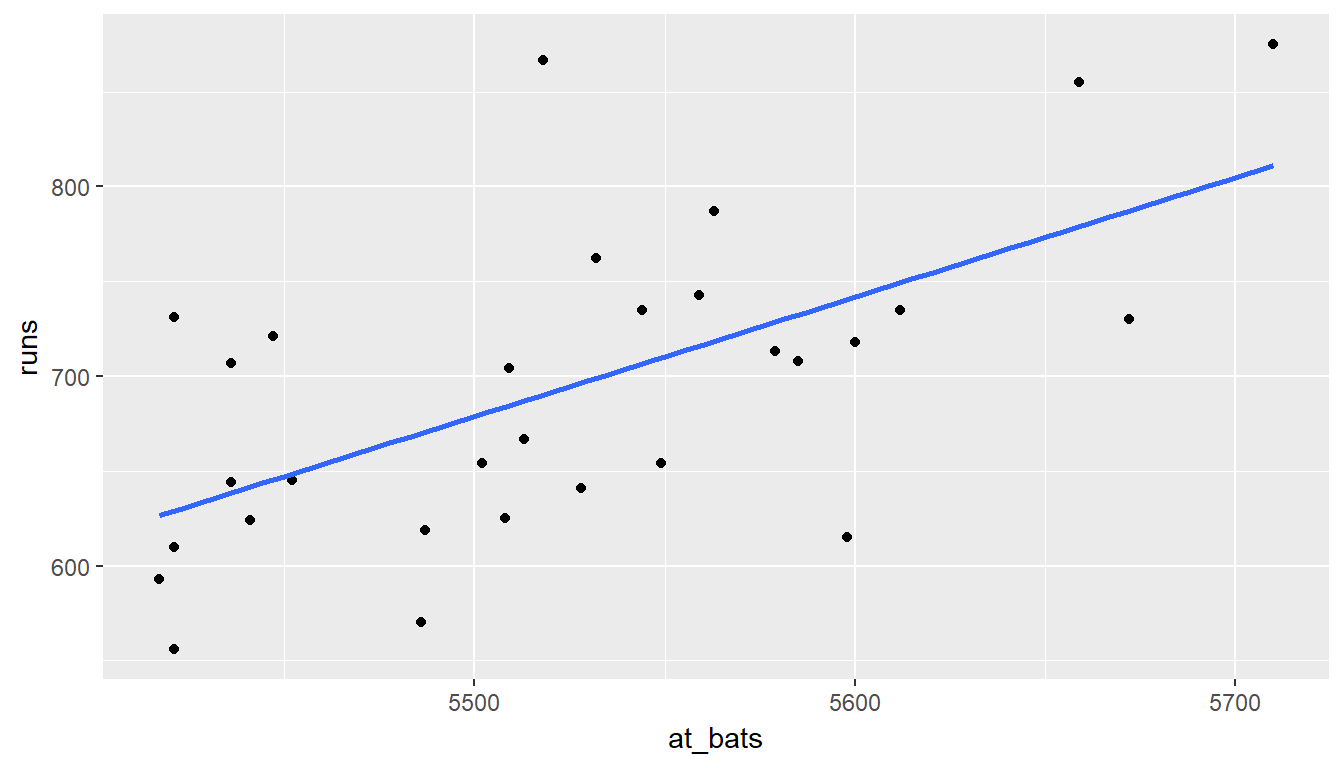Exercise: If you knew a team’s at_bats, would you be comfortable using a linear model to predict their number of runs?

answer: yes, as long as the number of at bats was between 5400 and 5700.

If the relationship looks linear, we can quantify the strength of the relationship with the correlation coefficient.

mlb11 %>%
summarise(cor(runs, at_bats))
## # A tibble: 1 x 1
##   cor(runs, at_bats)
##                  <dbl>
## 1             0.610627

## Sum of squared residuals

In this section you will use an interactive function to investigate what we mean by “sum of squared residuals”. You will need to run this function in your console, not in your markdown document. Running the function also requires that the mlb11 dataset is loaded in your environment.

Think back to the way that we described the distribution of a single variable. Recall that we discussed characteristics such as center, spread, and shape. It’s also useful to be able to describe the relationship of two numerical variables, such as runs and at_bats above.

1. Looking at your plot from the previous exercise, which of the following best describe the relationship between these two variables?
1. The relationship is negative, linear, and moderately strong. One of the potential outliers is a team with approximately 5520 at bats.
2. The relationship is positive, linear, and moderately strong. One of the potential outliers is a team with approximately 5520 at bats.
3. The relationship is positive, linear, and very weak. There are no outliers.
4. The relationship is positive, linear, and very weak. One of the potential outliers is a team with approximately 5520 at bats.

answer: The relationship is positive, linear, and moderately strong. One of the potential outliers is a team with approximately 5520 at bats.

Just as we used the mean and standard deviation to summarize a single variable, we can summarize the relationship between these two variables by finding the line that best follows their association. Use the following interactive function to select the line that you think does the best job of going through the cloud of points.

plot_ss(x = at_bats, y = runs, data = mlb11)

After running this command, you’ll be prompted to click two points on the plot to define a line. Once you’ve done that, the line you specified will be shown in black and the residuals in blue. Note that there are 30 residuals, one for each of the 30 observations. Recall that the residuals are the difference between the observed values and the values predicted by the line:

$e_i = y_i - \hat{y}_i$

The most common way to do linear regression is to select the line that minimizes the sum of squared residuals. To visualize the squared residuals, you can rerun the plot command and add the argument showSquares = TRUE.

plot_ss(x = at_bats, y = runs, data = mlb11, showSquares = TRUE)

Note that the output from the plot_ss function provides you with the slope and intercept of your line as well as the sum of squares.

Exercise: Using plot_ss, choose a line that does a good job of minimizing the sum of squares. Run the function several times. Report your smallest sum of squares.

answer: smallest sum of squares is 140071 ## The linear model

It is rather cumbersome to try to get the correct least squares line, i.e. the line that minimizes the sum of squared residuals, through trial and error. Instead we can use the lm function in R to fit the linear model (a.k.a. regression line).

m1 <- lm(runs ~ at_bats, data = mlb11)

The first argument in the function lm is a formula that takes the form y ~ x. Here it can be read that we want to make a linear model of runs as a function of at_bats. The second argument specifies that R should look in the mlb11 data frame to find the runs and at_bats variables.

The output of lm is an object that contains all of the information we need about the linear model that was just fit. We can access this information using the summary function.

summary(m1)
##
## Call:
## lm(formula = runs ~ at_bats, data = mlb11)
##
## Residuals:
##     Min      1Q  Median      3Q     Max
## -125.58  -47.05  -16.59   54.40  176.87
##
## Coefficients:
##               Estimate Std. Error t value Pr(>|t|)
## (Intercept) -2789.2429   853.6957  -3.267 0.002871 **
## at_bats         0.6305     0.1545   4.080 0.000339 ***
## ---
## Signif. codes:  0 '***' 0.001 '**' 0.01 '*' 0.05 '.' 0.1 ' ' 1
##
## Residual standard error: 66.47 on 28 degrees of freedom
## Multiple R-squared:  0.3729, Adjusted R-squared:  0.3505
## F-statistic: 16.65 on 1 and 28 DF,  p-value: 0.0003388

Let’s consider this output piece by piece. First, the formula used to describe the model is shown at the top. After the formula you find the five-number summary of the residuals. The “Coefficients” table shown next is key; its first column displays the linear model’s y-intercept and the coefficient of at_bats. With this table, we can write down the least squares regression line for the linear model:

$\hat{y} = -2789.2429 + 0.6305 \times at\_bats$

One last piece of information we will discuss from the summary output is the Multiple R-squared, or more simply, $$R^2$$. The $$R^2$$ value represents the proportion of variability in the response variable that is explained by the explanatory variable. For this model, 37.3% of the variability in runs is explained by at-bats.

1. Fit a new model that uses homeruns to predict runs. Using the estimates from the R output, write the equation of the regression line. What does the slope tell us in the context of the relationship between success of a team and its home runs?
1. For each additional home run, the model predicts 1.83 more runs, on average.
2. Each additional home run increases runs by 1.83.
3. For each additional home run, the model predicts 1.83 fewer runs, on average.
4. For each additional home run, the model predicts 415.24 more runs, on average.
5. For each additional home run, the model predicts 415.24 fewer runs, on average.
# type your code for Question 4 here, and Knit
m2 <- lm(runs ~ homeruns, data = mlb11)
summary(m2)
##
## Call:
## lm(formula = runs ~ homeruns, data = mlb11)
##
## Residuals:
##     Min      1Q  Median      3Q     Max
## -91.615 -33.410   3.231  24.292 104.631
##
## Coefficients:
##             Estimate Std. Error t value Pr(>|t|)
## (Intercept) 415.2389    41.6779   9.963 1.04e-10 ***
## homeruns      1.8345     0.2677   6.854 1.90e-07 ***
## ---
## Signif. codes:  0 '***' 0.001 '**' 0.01 '*' 0.05 '.' 0.1 ' ' 1
##
## Residual standard error: 51.29 on 28 degrees of freedom
## Multiple R-squared:  0.6266, Adjusted R-squared:  0.6132
## F-statistic: 46.98 on 1 and 28 DF,  p-value: 1.9e-07

answer: $\hat{y} = 415.2389+ 1.8345 \times homeruns$ For each additional home run, the model predicts 1.83 more runs, on average.

## Prediction and prediction errors

Let’s create a scatterplot with the least squares line for m1 laid on top.

ggplot(data = mlb11, aes(x = at_bats, y = runs)) +
geom_point() +
stat_smooth(method = "lm", se = FALSE)Here we are literally adding a layer on top of our plot. stat_smooth creates the line by fitting a linear model. It can also show us the standard error se associated with our line, but we’ll suppress that for now.

This line can be used to predict $$y$$ at any value of $$x$$. When predictions are made for values of $$x$$ that are beyond the range of the observed data, it is referred to as extrapolation and is not usually recommended. However, predictions made within the range of the data are more reliable. They’re also used to compute the residuals.

Exercise: If a team manager saw the least squares regression line and not the actual data, how many runs would he or she predict for a team with 5,579 at-bats? Is this an overestimate or an underestimate, and by how much?

-2789.2429 + 0.6305 * 5579
##  728.3166

To find the observed number of runs for the team with 5,579 at bats you can use the following:

mlb11 %>%
filter(at_bats == 5579) %>%
select(runs)

This code first filters for rows observation. at_bats is 5579, and then shows the value of the runs variable for that observation.

1. What is the residual for the prediction of runs for a team with 5,579 at-bats? Choose the closest answer.
1. -15.32
2. 15.32
3. 713
4. 5579
# type your code for Question 5 here, and Knit
713-(-2789.2429 + 0.6305 * 5579)
##  -15.3166

## Model diagnostics

To assess whether the linear model is reliable, we need to check for (1) linearity, (2) nearly normal residuals, and (3) constant variability.

Linearity: You already checked if the relationship between runs and at-bats is linear using a scatterplot. We should also verify this condition with a plot of the residuals vs. fitted (predicted) values.

ggplot(data = m1, aes(x = .fitted, y = .resid)) +
geom_point() +
geom_hline(yintercept = 0, linetype = "dashed") +
xlab("Fitted values") +
ylab("Residuals")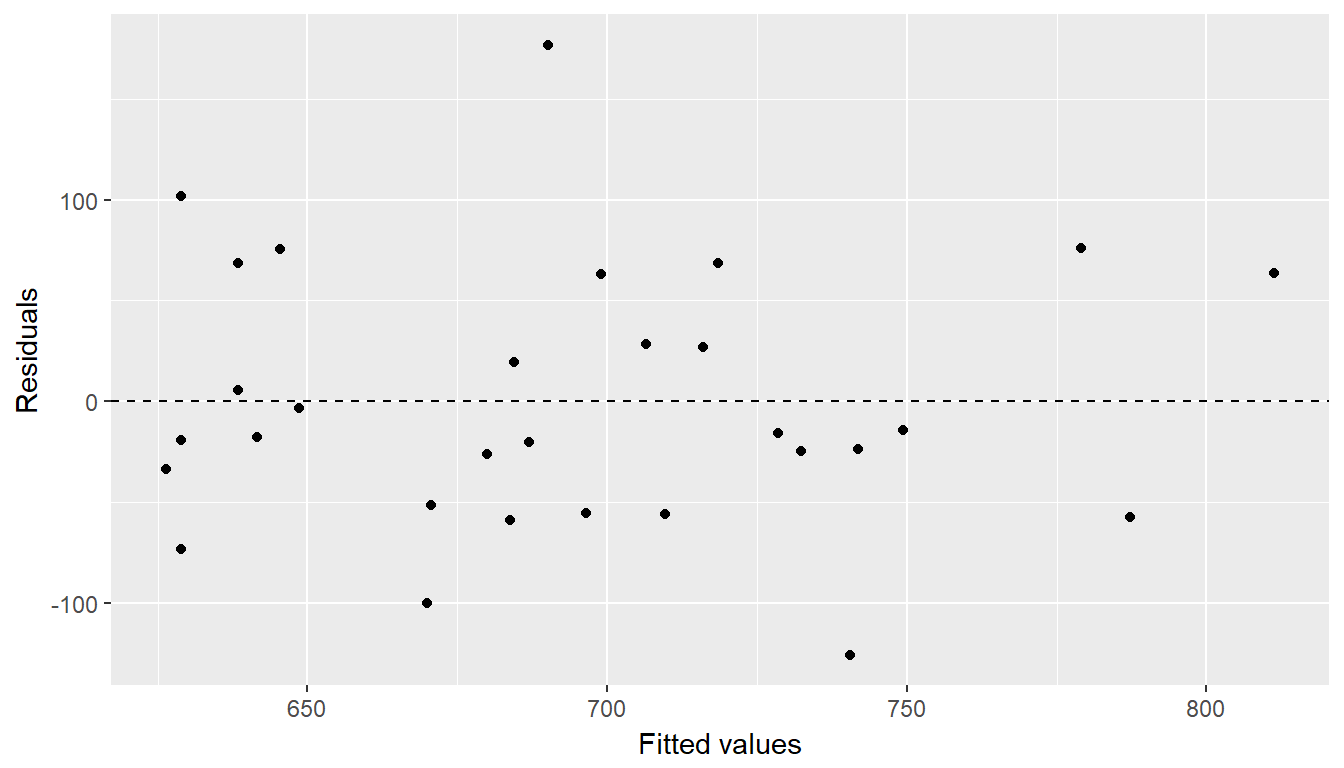Notice here that our model object m1 can also serve as a data set because stored within it are the fitted values ($$\hat{y}$$) and the residuals. Also note that we’re getting fancy with the code here. After creating the scatterplot on the first layer (first line of code), we overlay a horizontal dashed line at $$y = 0$$ (to help us check whether residuals are distributed around 0), and we also adjust the axis labels to be more informative.

1. Which of the following statements about the residual plot is false?
1. The residuals appear to be randomly distributed around 0.
2. The residuals show a curved pattern.
3. The plot is indicative of a linear relationship between runs and at-bats.
4. The team with a very high residual compared to the others appears to be an outlier.

answer: The residuals show a curved pattern.

Nearly normal residuals: To check this condition, we can look at a histogram

ggplot(data = m1, aes(x = .resid)) +
geom_histogram(binwidth = 25) +
xlab("Residuals")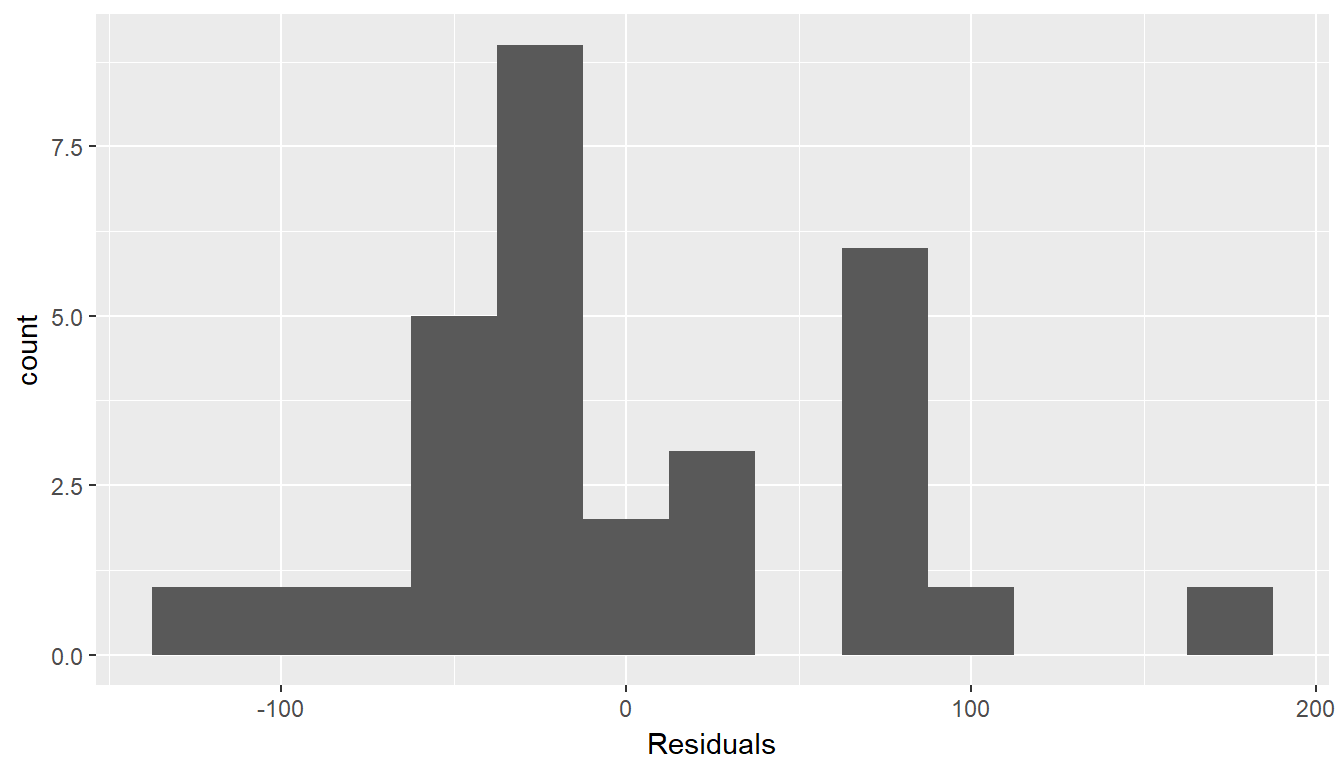or a normal probability plot of the residuals.

ggplot(data = m1, aes(sample = .resid)) +
stat_qq()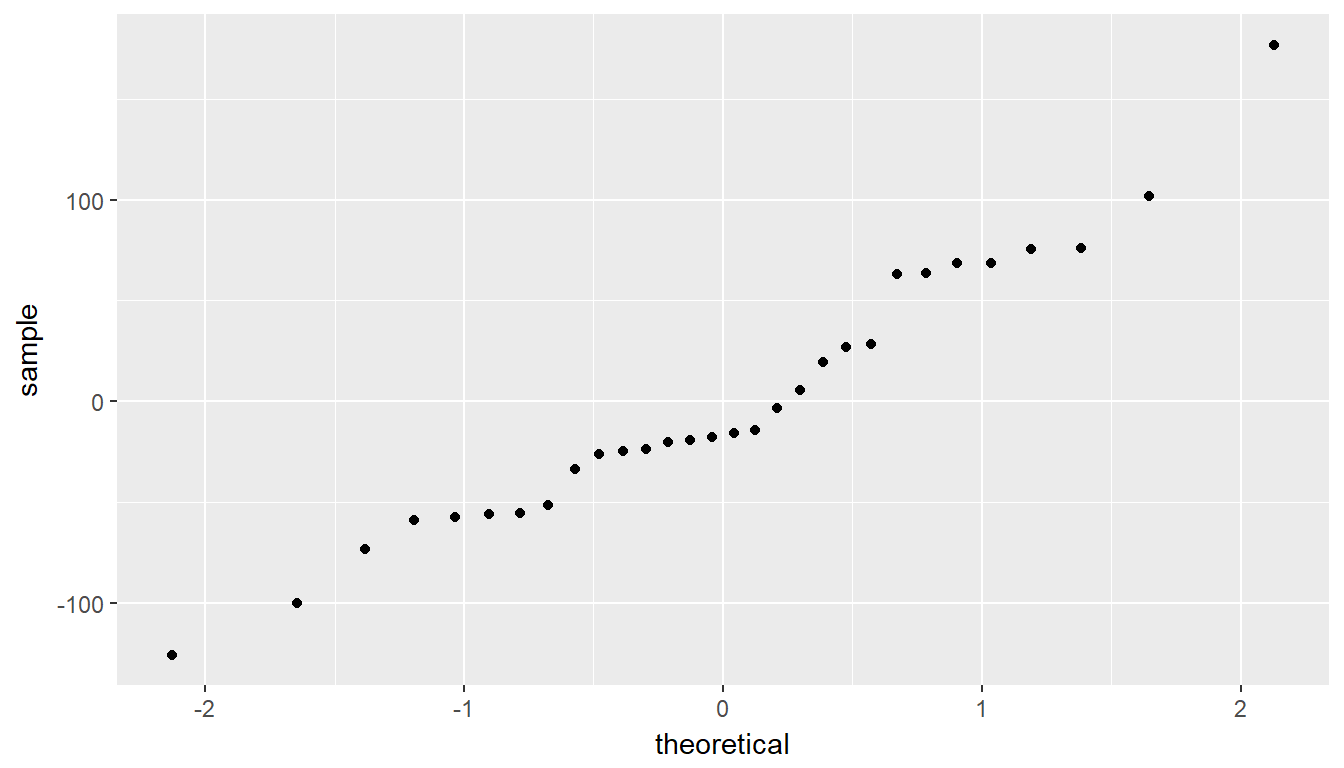Note that the syntax for making a normal probability plot is a bit different than what you’re used to seeing: we set sample equal to the residuals instead of x, and we set a statistical method qq, which stands for “quantile-quantile”, another name commonly used for normal probability plots.

1. Which of the following is true?
1. The residuals are extremely right skewed, hence the normal distribution of residuals condition is not met.
2. The residuals are extremely left skewed, hence the normal distribution of residuals condition is not met.
3. The residuals are perfectly symmetric, hence the normal distribution of residuals condition is met.
4. The residuals are fairly symmetric, with only a slightly longer tail on the right, hence it would be appropriate to deem the the normal distribution of residuals condition met.

answer: The residuals are fairly symmetric, with only a slightly longer tail on the right, hence it would be appropriate to deem the the normal distribution of residuals condition met.

Constant variability:

1. Based on the residuals plot from earlier, the constant variability condition appears to be met.
1. True
2. False

Exercise: Choose another one of the seven traditional variables from mlb11 besides at_bats that you think might be a good predictor of runs. Produce a scatterplot of the two variables and fit a linear model. At a glance, does there seem to be a linear relationship?

# type your code for the Exercise here, and Knit
ggplot(data = mlb11, aes(x = wins, y = runs)) +
geom_point() +
stat_smooth(method = "lm", se = FALSE)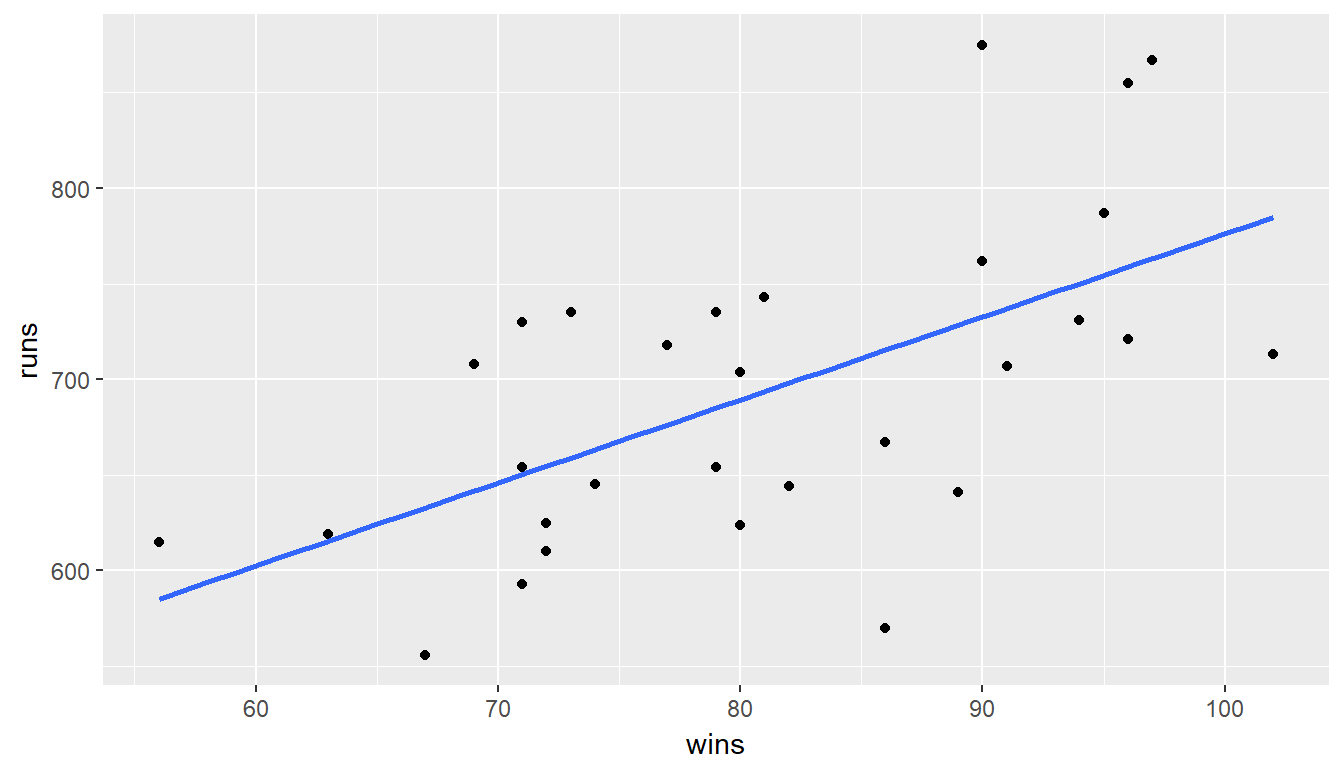answer: there does appear to be a linear relationship.

Exercise: How does this relationship compare to the relationship between runs and at_bats? Use the R$$^2$$ values from the two model summaries to compare. Does your variable seem to predict runs better than at_bats? How can you tell?

# type your code for the Exercise here, and Knit
summary(m1)
##
## Call:
## lm(formula = runs ~ at_bats, data = mlb11)
##
## Residuals:
##     Min      1Q  Median      3Q     Max
## -125.58  -47.05  -16.59   54.40  176.87
##
## Coefficients:
##               Estimate Std. Error t value Pr(>|t|)
## (Intercept) -2789.2429   853.6957  -3.267 0.002871 **
## at_bats         0.6305     0.1545   4.080 0.000339 ***
## ---
## Signif. codes:  0 '***' 0.001 '**' 0.01 '*' 0.05 '.' 0.1 ' ' 1
##
## Residual standard error: 66.47 on 28 degrees of freedom
## Multiple R-squared:  0.3729, Adjusted R-squared:  0.3505
## F-statistic: 16.65 on 1 and 28 DF,  p-value: 0.0003388
summary(m2)
##
## Call:
## lm(formula = runs ~ homeruns, data = mlb11)
##
## Residuals:
##     Min      1Q  Median      3Q     Max
## -91.615 -33.410   3.231  24.292 104.631
##
## Coefficients:
##             Estimate Std. Error t value Pr(>|t|)
## (Intercept) 415.2389    41.6779   9.963 1.04e-10 ***
## homeruns      1.8345     0.2677   6.854 1.90e-07 ***
## ---
## Signif. codes:  0 '***' 0.001 '**' 0.01 '*' 0.05 '.' 0.1 ' ' 1
##
## Residual standard error: 51.29 on 28 degrees of freedom
## Multiple R-squared:  0.6266, Adjusted R-squared:  0.6132
## F-statistic: 46.98 on 1 and 28 DF,  p-value: 1.9e-07

answer: the R2 is slightly smaller than that associated with at_bats.

1. Now that you can summarize the linear relationship between two variables, investigate the relationships between runs and each of the other five traditional variables. Which variable best predicts runs? Support your conclusion using the graphical and numerical methods we’ve discussed.
1. at bats
2. hits
3. wins
4. batting average
# type your code for Question 9 here, and Knit
ggplot(data = mlb11, aes(x = at_bats, y = runs)) +
geom_point() +
geom_smooth(method = "lm", se = FALSE)ggplot(data = mlb11, aes(x = hits, y = runs)) +
geom_point() +
geom_smooth(method = "lm", se = FALSE)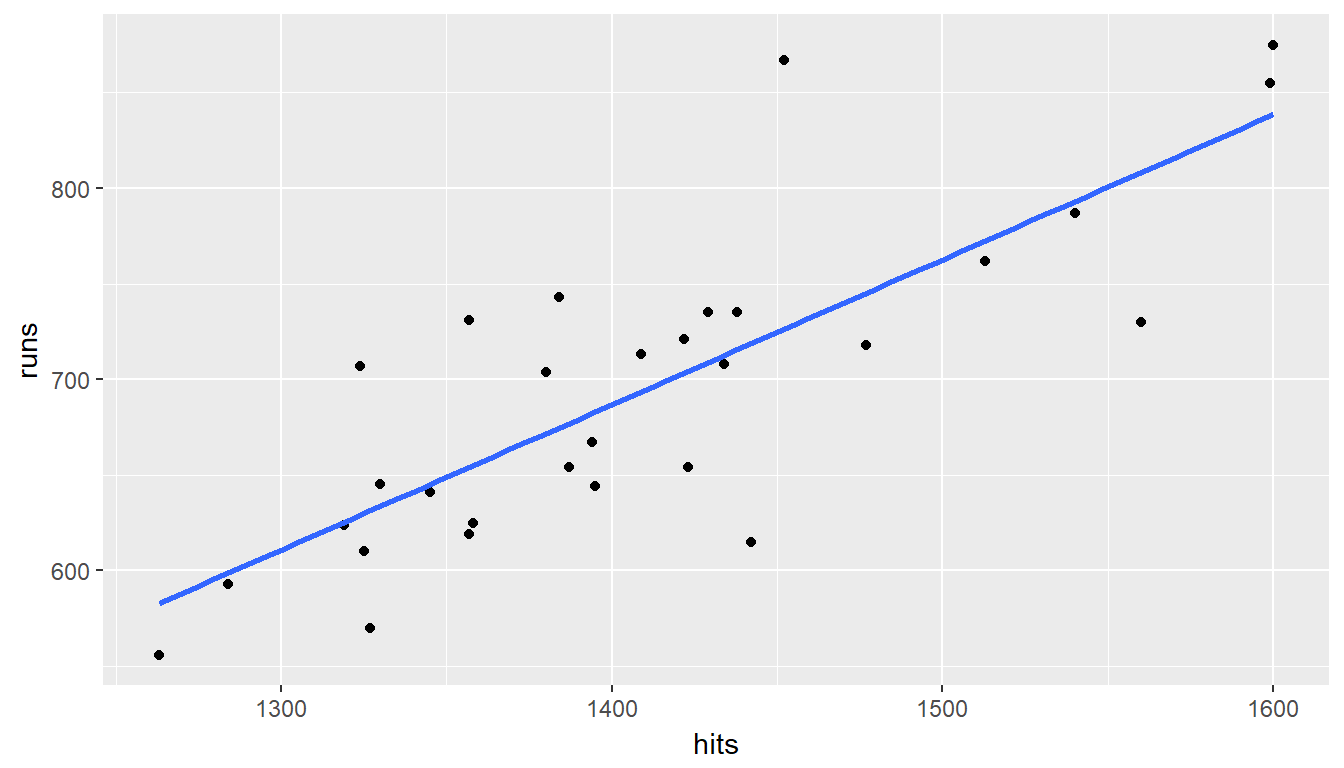ggplot(data = mlb11, aes(x = homeruns, y = runs)) +
geom_point() +
geom_smooth(method = "lm", se = FALSE)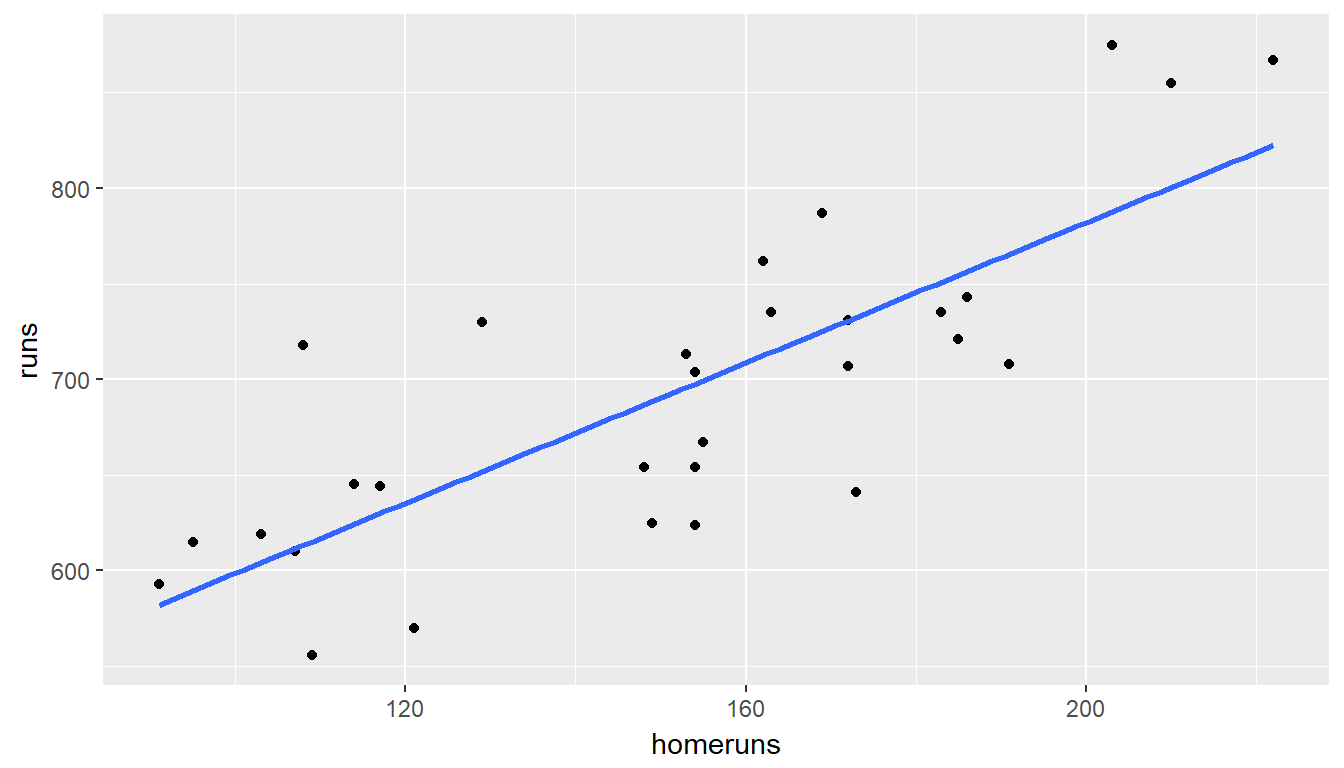ggplot(data = mlb11, aes(x = bat_avg, y = runs)) +
geom_point() +
geom_smooth(method = "lm", se = FALSE)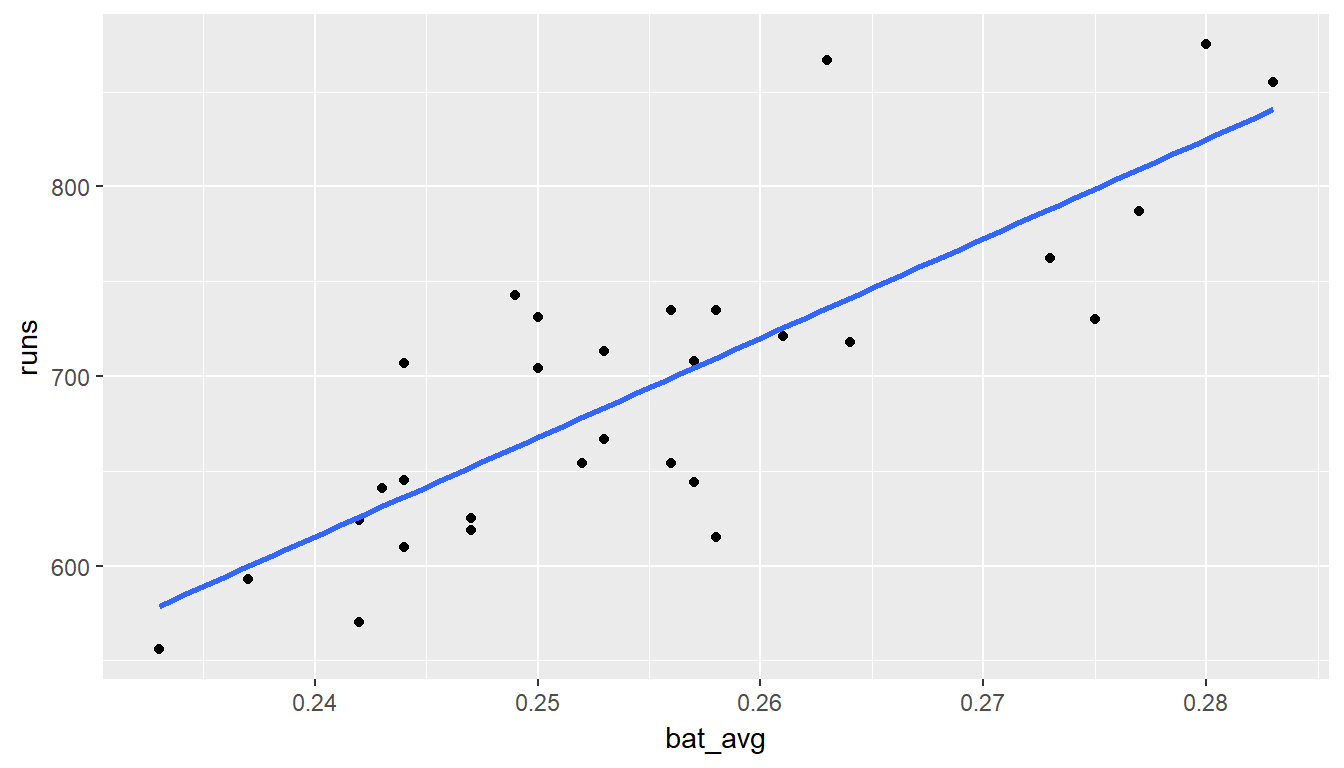ggplot(data = mlb11, aes(x = strikeouts, y = runs)) +
geom_point() +
geom_smooth(method = "lm", se = FALSE)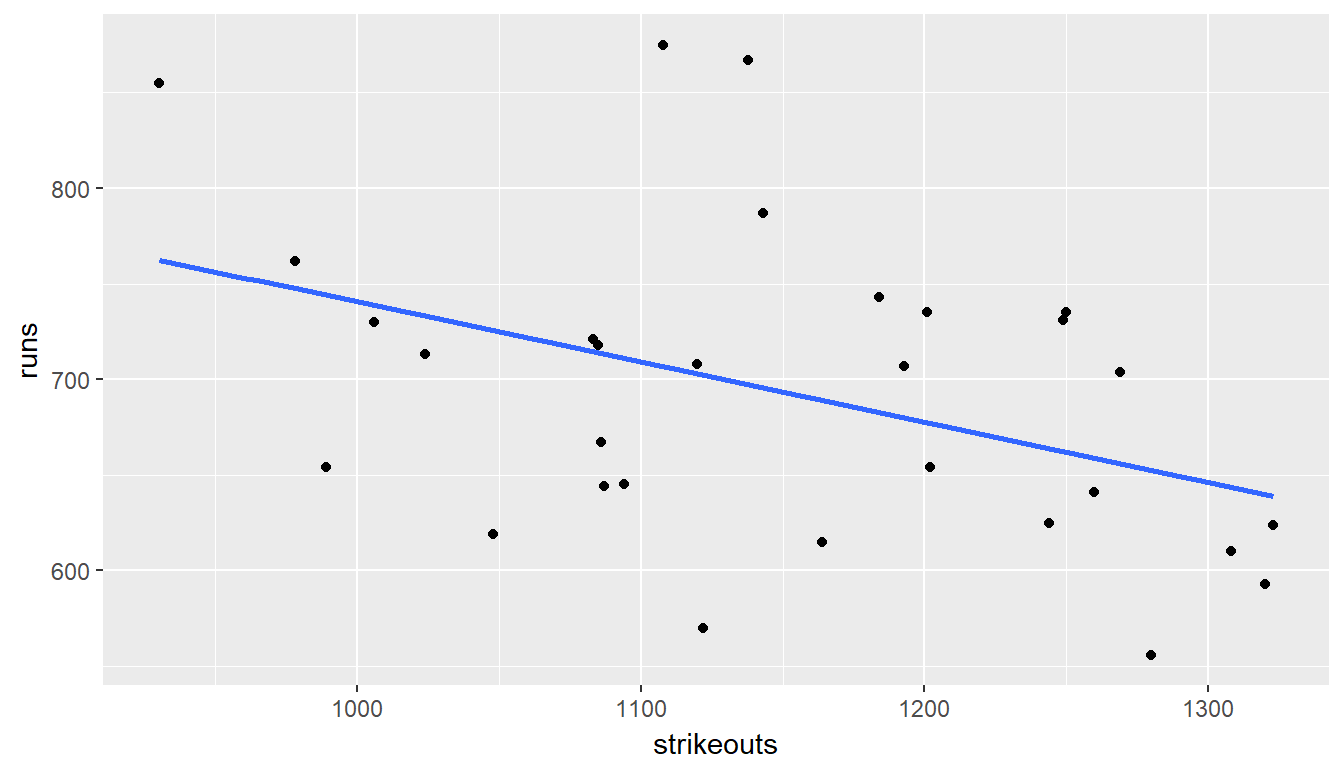ggplot(data = mlb11, aes(x = stolen_bases, y = runs)) +
geom_point() +
geom_smooth(method = "lm", se = FALSE)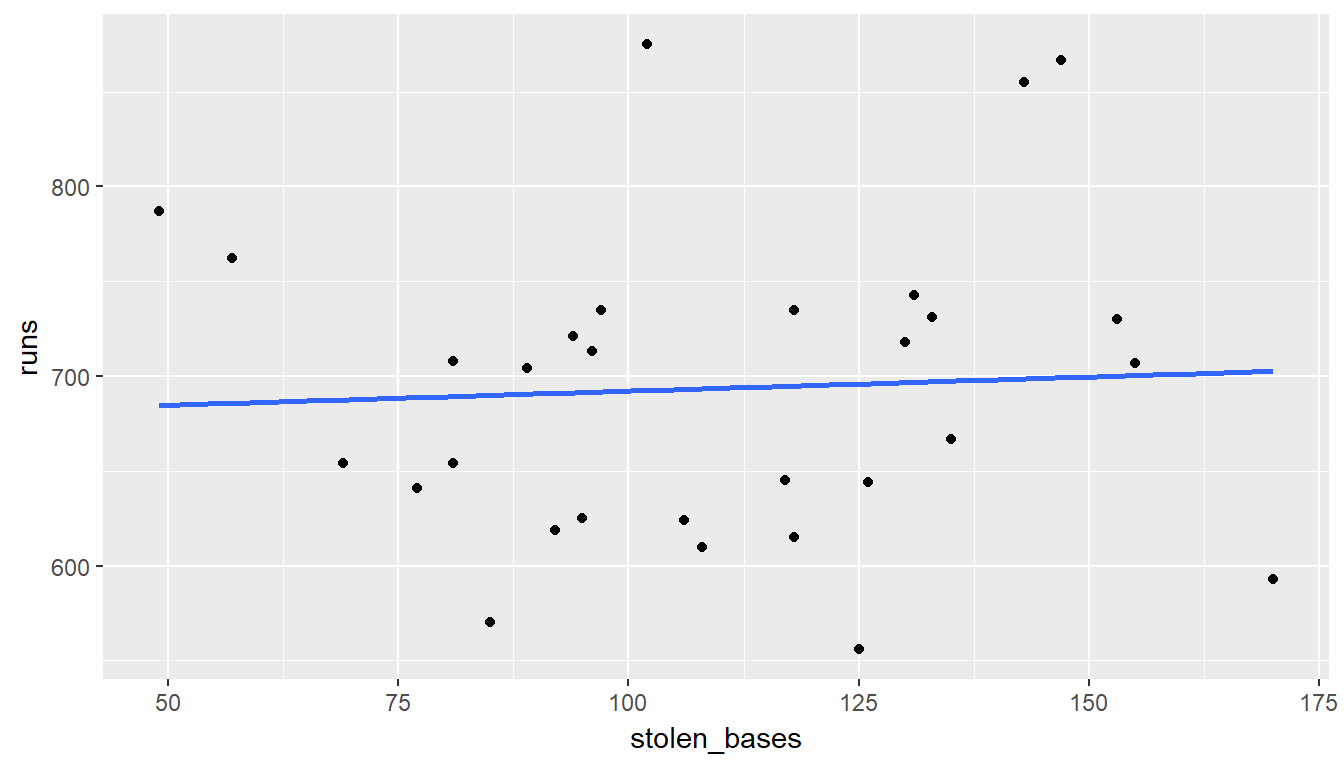m_at_bats <- lm(runs ~ at_bats, data = mlb11)
m_hits <- lm(runs ~ hits, data = mlb11)
m_homeruns <- lm(runs ~ homeruns, data = mlb11)
m_bat_avg <- lm(runs ~ bat_avg, data = mlb11)
m_strikeouts <- lm(runs ~ strikeouts, data = mlb11)
m_stolen_bases <- lm(runs ~ stolen_bases, data = mlb11)
summary(m_at_bats)
##
## Call:
## lm(formula = runs ~ at_bats, data = mlb11)
##
## Residuals:
##     Min      1Q  Median      3Q     Max
## -125.58  -47.05  -16.59   54.40  176.87
##
## Coefficients:
##               Estimate Std. Error t value Pr(>|t|)
## (Intercept) -2789.2429   853.6957  -3.267 0.002871 **
## at_bats         0.6305     0.1545   4.080 0.000339 ***
## ---
## Signif. codes:  0 '***' 0.001 '**' 0.01 '*' 0.05 '.' 0.1 ' ' 1
##
## Residual standard error: 66.47 on 28 degrees of freedom
## Multiple R-squared:  0.3729, Adjusted R-squared:  0.3505
## F-statistic: 16.65 on 1 and 28 DF,  p-value: 0.0003388
summary(m_hits)
##
## Call:
## lm(formula = runs ~ hits, data = mlb11)
##
## Residuals:
##      Min       1Q   Median       3Q      Max
## -103.718  -27.179   -5.233   19.322  140.693
##
## Coefficients:
##              Estimate Std. Error t value Pr(>|t|)
## (Intercept) -375.5600   151.1806  -2.484   0.0192 *
## hits           0.7589     0.1071   7.085 1.04e-07 ***
## ---
## Signif. codes:  0 '***' 0.001 '**' 0.01 '*' 0.05 '.' 0.1 ' ' 1
##
## Residual standard error: 50.23 on 28 degrees of freedom
## Multiple R-squared:  0.6419, Adjusted R-squared:  0.6292
## F-statistic:  50.2 on 1 and 28 DF,  p-value: 1.043e-07
summary(m_homeruns)
##
## Call:
## lm(formula = runs ~ homeruns, data = mlb11)
##
## Residuals:
##     Min      1Q  Median      3Q     Max
## -91.615 -33.410   3.231  24.292 104.631
##
## Coefficients:
##             Estimate Std. Error t value Pr(>|t|)
## (Intercept) 415.2389    41.6779   9.963 1.04e-10 ***
## homeruns      1.8345     0.2677   6.854 1.90e-07 ***
## ---
## Signif. codes:  0 '***' 0.001 '**' 0.01 '*' 0.05 '.' 0.1 ' ' 1
##
## Residual standard error: 51.29 on 28 degrees of freedom
## Multiple R-squared:  0.6266, Adjusted R-squared:  0.6132
## F-statistic: 46.98 on 1 and 28 DF,  p-value: 1.9e-07
summary(m_bat_avg)
##
## Call:
## lm(formula = runs ~ bat_avg, data = mlb11)
##
## Residuals:
##     Min      1Q  Median      3Q     Max
## -94.676 -26.303  -5.496  28.482 131.113
##
## Coefficients:
##             Estimate Std. Error t value Pr(>|t|)
## (Intercept)   -642.8      183.1  -3.511  0.00153 **
## bat_avg       5242.2      717.3   7.308 5.88e-08 ***
## ---
## Signif. codes:  0 '***' 0.001 '**' 0.01 '*' 0.05 '.' 0.1 ' ' 1
##
## Residual standard error: 49.23 on 28 degrees of freedom
## Multiple R-squared:  0.6561, Adjusted R-squared:  0.6438
## F-statistic: 53.41 on 1 and 28 DF,  p-value: 5.877e-08
summary(m_strikeouts)
##
## Call:
## lm(formula = runs ~ strikeouts, data = mlb11)
##
## Residuals:
##     Min      1Q  Median      3Q     Max
## -132.27  -46.95  -11.92   55.14  169.76
##
## Coefficients:
##              Estimate Std. Error t value Pr(>|t|)
## (Intercept) 1054.7342   151.7890   6.949 1.49e-07 ***
## strikeouts    -0.3141     0.1315  -2.389   0.0239 *
## ---
## Signif. codes:  0 '***' 0.001 '**' 0.01 '*' 0.05 '.' 0.1 ' ' 1
##
## Residual standard error: 76.5 on 28 degrees of freedom
## Multiple R-squared:  0.1694, Adjusted R-squared:  0.1397
## F-statistic: 5.709 on 1 and 28 DF,  p-value: 0.02386
summary(m_stolen_bases)
##
## Call:
## lm(formula = runs ~ stolen_bases, data = mlb11)
##
## Residuals:
##     Min      1Q  Median      3Q     Max
## -139.94  -62.87   10.01   38.54  182.49
##
## Coefficients:
##              Estimate Std. Error t value Pr(>|t|)
## (Intercept)  677.3074    58.9751  11.485 4.17e-12 ***
## stolen_bases   0.1491     0.5211   0.286    0.777
## ---
## Signif. codes:  0 '***' 0.001 '**' 0.01 '*' 0.05 '.' 0.1 ' ' 1
##
## Residual standard error: 83.82 on 28 degrees of freedom
## Multiple R-squared:  0.002914,   Adjusted R-squared:  -0.0327
## F-statistic: 0.08183 on 1 and 28 DF,  p-value: 0.7769

1. Now examine the three newer variables. These are the statistics used by the author of Moneyball to predict a teams success. In general, are they more or less effective at predicting runs that the old variables? Explain using appropriate graphical and numerical evidence. Of all ten variables we’ve analyzed, which seems to be the best predictor of runs?
1. on-base plus slugging (new_obs)
2. slugging percentage (new_slug)
3. on-base percentage (new_onbase)
# type your code for Question 10 here, and Knit
ggplot(data = mlb11, aes(x = new_onbase, y = runs)) +
geom_point() +
geom_smooth(method = "lm", se = FALSE)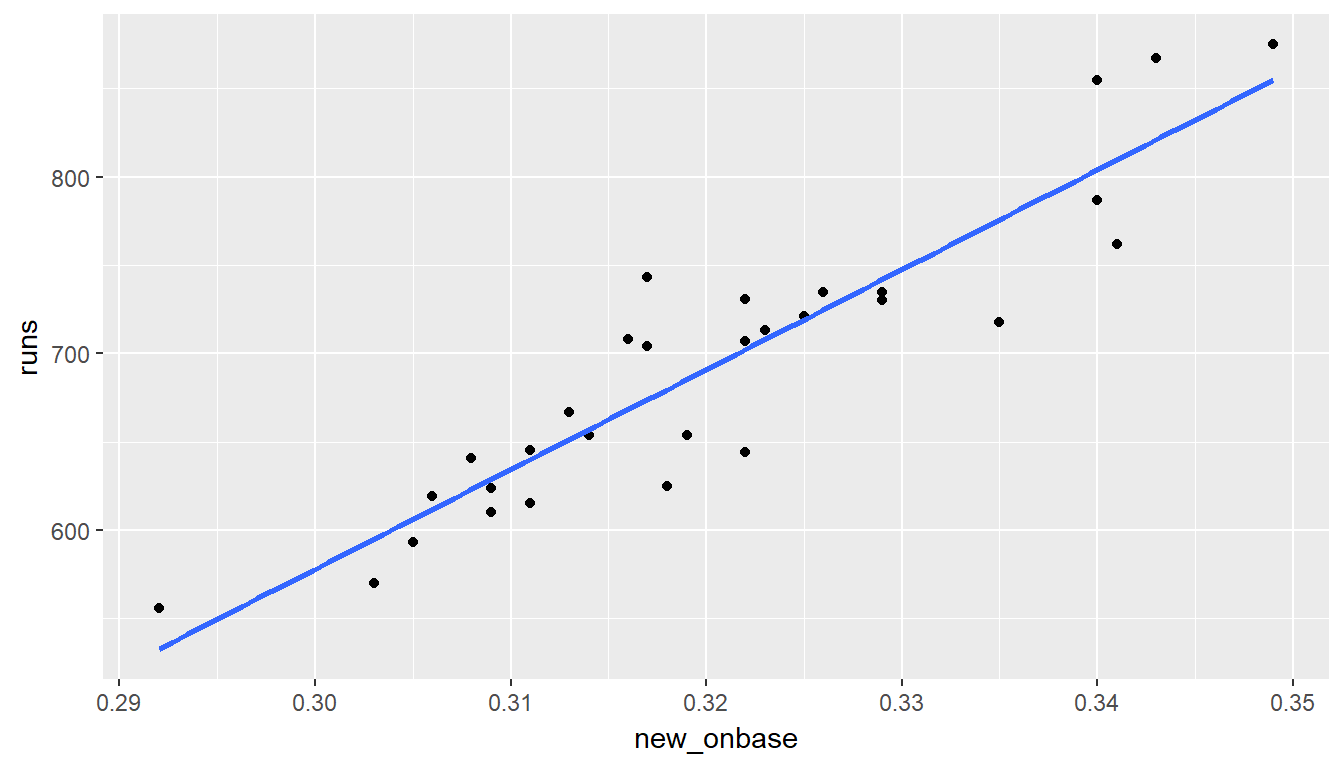ggplot(data = mlb11, aes(x = new_slug, y = runs)) +
geom_point() +
geom_smooth(method = "lm", se = FALSE)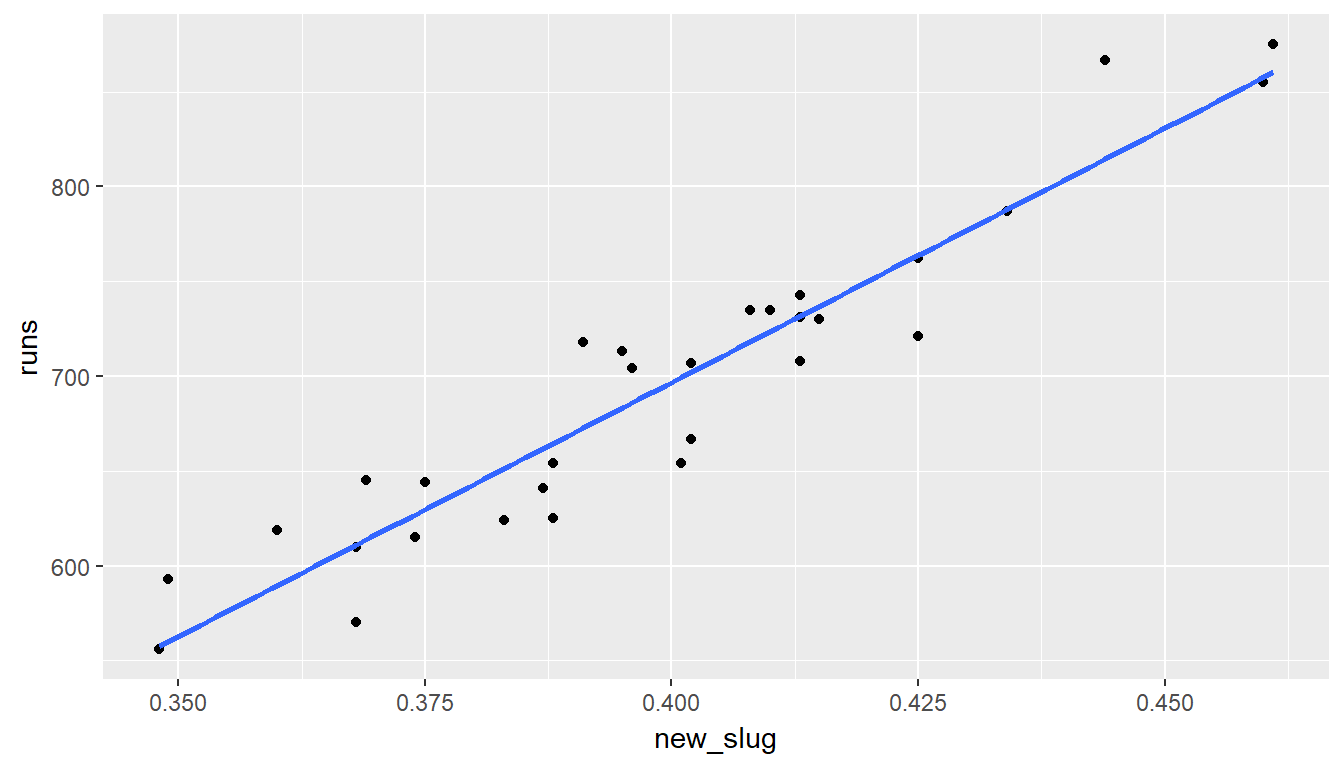ggplot(data = mlb11, aes(x = new_obs, y = runs)) +
geom_point() +
geom_smooth(method = "lm", se = FALSE)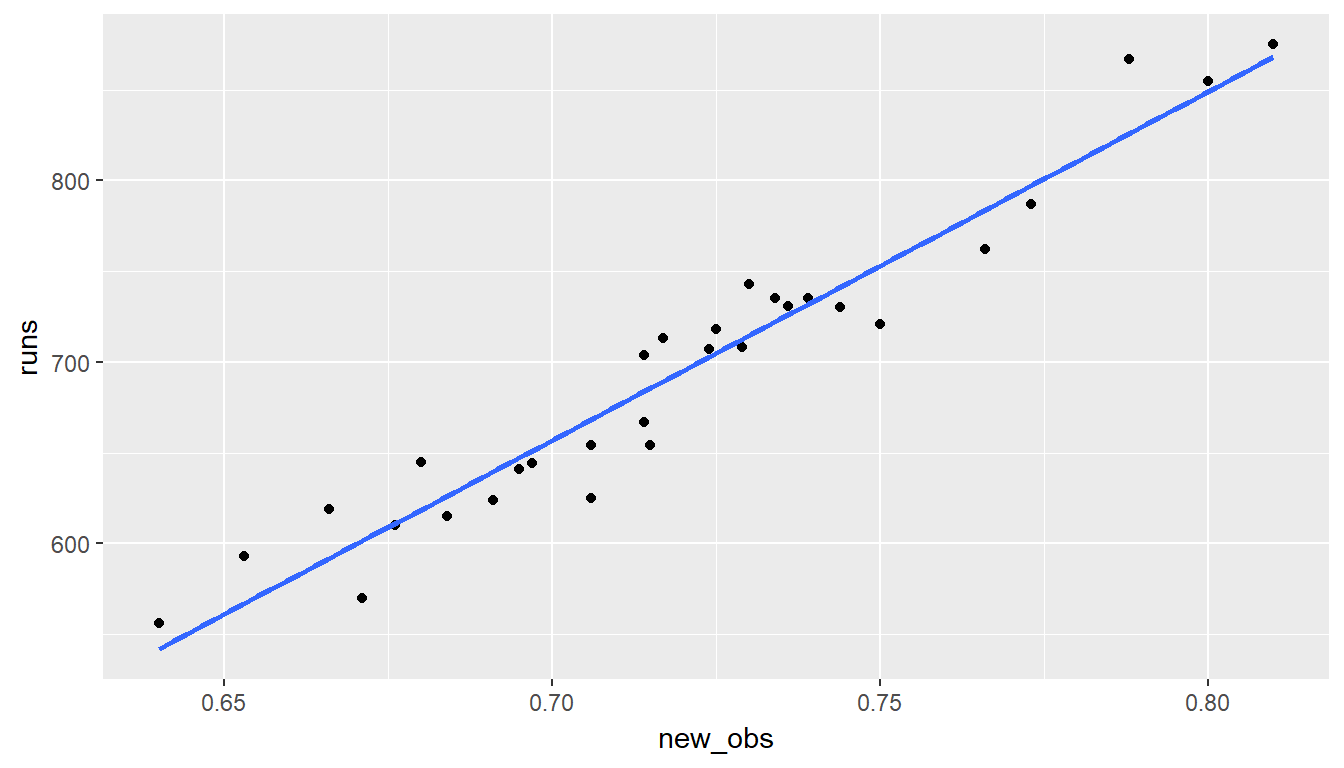m_new_onbase <- lm(runs ~ new_onbase, data = mlb11)
m_new_slug <- lm(runs ~ new_slug, data = mlb11)
m_new_obs <- lm(runs ~ new_obs, data = mlb11)
summary(m_new_onbase)
##
## Call:
## lm(formula = runs ~ new_onbase, data = mlb11)
##
## Residuals:
##     Min      1Q  Median      3Q     Max
## -58.270 -18.335   3.249  19.520  69.002
##
## Coefficients:
##             Estimate Std. Error t value Pr(>|t|)
## (Intercept)  -1118.4      144.5  -7.741 1.97e-08 ***
## new_onbase    5654.3      450.5  12.552 5.12e-13 ***
## ---
## Signif. codes:  0 '***' 0.001 '**' 0.01 '*' 0.05 '.' 0.1 ' ' 1
##
## Residual standard error: 32.61 on 28 degrees of freedom
## Multiple R-squared:  0.8491, Adjusted R-squared:  0.8437
## F-statistic: 157.6 on 1 and 28 DF,  p-value: 5.116e-13
summary(m_new_slug)
##
## Call:
## lm(formula = runs ~ new_slug, data = mlb11)
##
## Residuals:
##    Min     1Q Median     3Q    Max
## -45.41 -18.66  -0.91  16.29  52.29
##
## Coefficients:
##             Estimate Std. Error t value Pr(>|t|)
## (Intercept)  -375.80      68.71   -5.47 7.70e-06 ***
## new_slug     2681.33     171.83   15.61 2.42e-15 ***
## ---
## Signif. codes:  0 '***' 0.001 '**' 0.01 '*' 0.05 '.' 0.1 ' ' 1
##
## Residual standard error: 26.96 on 28 degrees of freedom
## Multiple R-squared:  0.8969, Adjusted R-squared:  0.8932
## F-statistic: 243.5 on 1 and 28 DF,  p-value: 2.42e-15
summary(m_new_obs)
##
## Call:
## lm(formula = runs ~ new_obs, data = mlb11)
##
## Residuals:
##     Min      1Q  Median      3Q     Max
## -43.456 -13.690   1.165  13.935  41.156
##
## Coefficients:
##             Estimate Std. Error t value Pr(>|t|)
## (Intercept)  -686.61      68.93  -9.962 1.05e-10 ***
## new_obs      1919.36      95.70  20.057  < 2e-16 ***
## ---
## Signif. codes:  0 '***' 0.001 '**' 0.01 '*' 0.05 '.' 0.1 ' ' 1
##
## Residual standard error: 21.41 on 28 degrees of freedom
## Multiple R-squared:  0.9349, Adjusted R-squared:  0.9326
## F-statistic: 402.3 on 1 and 28 DF,  p-value: < 2.2e-16

answer: on-base plus slugging (new_obs)

Exercise: Check the model diagnostics for the regression model with the variable you decided was the best predictor for runs.

# type your code for the Exercise here, and Knit
m3 <- lm(runs ~ new_obs, data = mlb11)
ggplot(data = m3, aes(x = .fitted, y = .resid)) +
geom_point() +
geom_hline(yintercept = 0, linetype = "dashed") +
xlab("Fitted values") +
ylab("Residuals")`